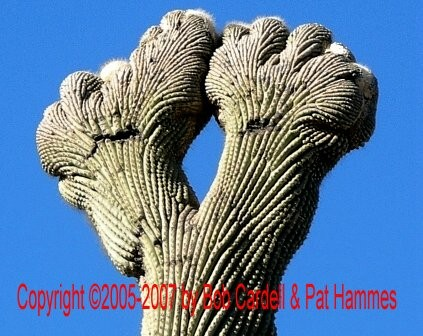"Lizard Man Praying"

View from the EastC0268 Photo courtesy of Bob Cardell & Pat Hammes

Pima,_Co.,
AZ. g h(w+w)/4
=c(g+h+c)
= xxx Lizard Man Praying:
Bob Cardell &
Pat Hammes
1-16
2006(w+h+d)
=? Standing
?g h(w+w)/4
=c(g+h+c)
=xxx ??(w+h+d)
=? ?
?g h(w+w)/4
=c(g+h+c)
=xxx ??(w+h+d)
=? ?